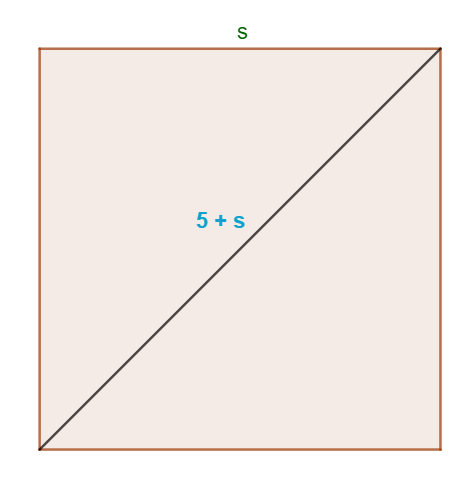### Math Notes

Subjects

#### Algebra Solutions

##### Topics || Problems

Find the side of a square whose diagonal is 5 feet longer than a side.$$d = 5 + s$$

Using the Pythagorean Theorem the diagonal is equal to $$s \sqrt{2}$$

$$d= 5 +s =s \sqrt{2}$$

$$5 = s \sqrt{2} -s$$

$$5 = s ( \sqrt{2} -1)$$

$$s = 5+5\sqrt{2}$$